# Sorting 2D Shapes

Sorting 2D Shapes#### Sorting Shapes with a Carroll Diagram• A Carroll Diagram is used to sort shapes by placing them into the boxes that are in line with their properties.
• We will sort this isosceles triangle using the Carroll diagram.
• The triangle has 3 corners.
• Therefore it will belong in the column that says ‘4 corners or less’.
• All of its sides are not the same size. The base is smaller than the other two sides.
• Therefore it belongs in the row that says ‘sides of different lengths’.
• We put the triangle in the box that is in line with these two properties.

A Carroll Diagram is a table of mathematical properties.

Each shape is placed into the box that is in line with its properties.

#### Sorting Shapes with a Venn Diagram• This Venn diagram is used to sort shapes by placing them into the circles which represent their properties.
• The left circle will contain shapes that contain a right angle.
• The right circle will contain shapes that have sides that are the same length.
• All of the angles on a square are right angles.
• Since it contains a right angle it needs to be placed somewhere inside the left circle.
• All of a square’s sides are the same length.
• Because all of the sides are the same length, the square must also be placed inside the right circle.
• We need to place the shape inside both circles.
• The square needs to be placed in the overlapping area since it contains the properties of both circles.

On a Venn Diagram, each circle represents a different property.

The shapes are placed inside the circles which represent the properties that the shape has.• The left circle on the Venn diagram is for shapes that contain a right angle.
• The right circle on the Venn diagram is for shapes in which every side is the same length.
• The square contains a right angle and has sides that are the same length.
• It belongs in both circles and so, it is sorted into the overlapping area in the middle.
• The rhombus (diamond) does not contain any right angles but does have sides that are the same length.
• It belongs in the right circle but not in the left circle.
• The pentagon does not contain a right angle but its sides are all the same length.
• It belongs in the right circle but not the left circle.

• This triangle contains a right angle, however its sides are not all the same length.
• It is sorted into the left circle but not the right circle.
• This trapezium does not contain a right angle.and its sides are not all the same size.
• It does not belong in either circle and it is left outside.
• This octagon does not contain a right angle but all of its sides are the same size.
• It is placed in the right circle but not the left circle of the Venn diagram.Supporting Lessons

#### Sorting 2D Shapes Accompanying Activity Sheets# How to Sort 2D Shapes using a Venn or Carroll Diagram

## Sorting Shapes by their Properties

Sorting shapes by their attributes is a skill that is learnt at late KS1/ early KS2 (first to third grade) after learning the names and properties of common shapes.

We can list the properties of shapes using a table. There are many shape properties and many shapes and we will look at some in this example.

We will first sort these shapes to decide whether they contain straight sides.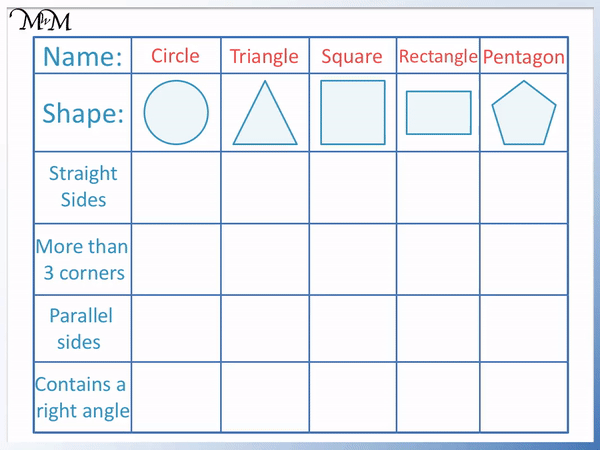• A circle does not have straight sides – it as curved sides.
• A triangle has 3 straight sides.
• A square has 4 straight sides.
• A rectangle has 4 straight sides.
• A pentagon has 5 straight sides.

We will now count the corners of these shapes to see if they have more than 3 corners.

Remember we need more than 3 corners to tick the shapes that have this property. When teaching sorting shapes, it is a common mistake to include shapes that have exactly 3 corners, which is not correct.

3 corners is not the same as more than 3 corners.• A circle has 0 corners, which is not more than 3.
• A triangle has 3 corners, which is not more than 3.
• A square has 4 corners, which is more than 3.
• A rectangle has 4 corners, which is more than 3.
• A pentagon has 5 corners, which is more than 3.

We will now sort the shapes by whether they contain parallel sides.Parallel sides are sides that face in exactly the same direction.• A circle only has 1 side and so, it does not contain parallel sides.
• A triangle does not have parallel sides.
• A square has 2 pairs of parallel sides. The top and bottom sides are parallel and the left and right sides are parallel.
• A rectangle has 2 pairs of parallel sides. The top and bottom sides are parallel and the left and right sides are parallel.
• A pentagon does not have parallel sides.

We will now sort the shapes by whether they contain a right angle.

A right angle is exactly 90 degrees. It looks like a ‘capital L’ shape.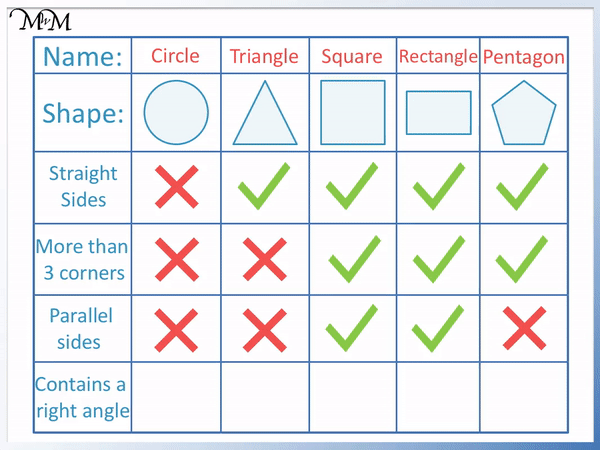• A circle does not contain a right angle.
• A triangle does not contain a right angle.
• A square contains right angles. All of its angles are right angles.
• A rectangle contains right angles. All of its angles are right angles..
• A pentagon does not contain any right angles.

Shapes are most commonly sorted using both a Venn diagram or a Carroll diagram. A Carroll diagram is different from a Venn diagram in that in a Carroll diagram objects are put into a table, whereas on a Venn Diagram objects are put into circles. On a Venn diagram, each circle is a different property and each object can be in more than one circle. However, on a Carroll diagram, the object can only go in one particular grid, in line with two of its properties.

## Sorting Shapes on a Carroll Diagram

A Carroll diagram is a table that can be used for sorting shapes by their attributes. The shapes will be placed inside the table in line with the properties in the rows and columns that describe it.

To sort shapes using a Carroll Diagram use these steps:

• Read the properties at the top of each column and decide which column applies.
• Read the properties on the left of each row and decide which row applies.
• Place the shape inside the box that lines up with the chosen column and the chosen row.

Here is an example of sorting shapes with a Carroll diagram. We have an isosceles triangle. It has 3 corners and its two sloping sides are a different length to its base side.

The triangle can only go in one of the four boxes shown in the Carroll diagram.

We first read the column properties. We have the choice of ‘4 corners or less’ or ‘more than 4 corners’.The triangle has 3 corners which is ‘4 corners or less’. It needs to be sorted into the left column.

We now need to decide if it belongs in the top-left box or the bottom-left box.

We have a choice of ‘sides the same length’ or ‘sides of different lengths’.

Even though this triangle has 2 sides that are the same length, this is not enough to say that every side is the same length. The base side is smaller than the other two.

We must sort the triangle into the row for the property ‘sides of different lengths’.

We place the shape in the box that lines up with the properties that the shape has. It needs to go in the left column because it has ‘4 corners or less’ and it needs to go in the bottom row because it has ‘sides of different lengths’.

The isosceles triangle is sorted into the bottom-left box of the Carroll diagram.

We will now arrange the rest of the shapes in the Carroll diagram.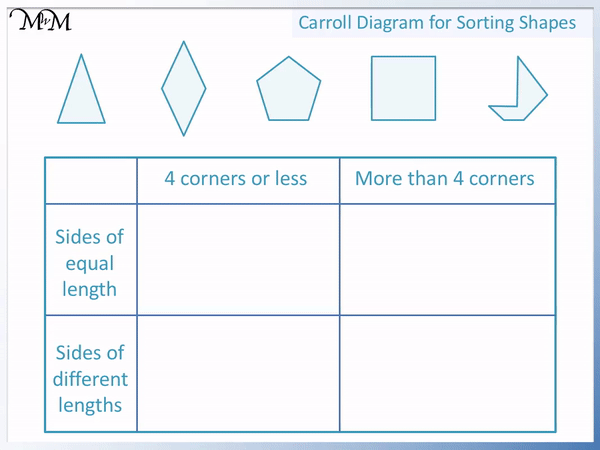• The rhombus (diamond) contains 4 corners and has sides that are all of equal length.
• The pentagon has 5 corners, which is ‘4 or more’ corners. It also has sides that are all equal in length.
• The square contains 4 corners and has sides that are equal in length.
• A rectangle contains right angles. All of its angles are right angles.
• The final shape has 6 corners, which is ‘4 or more’ corners. Its sides are not equal in length.

Here is another example of sorting shapes on a Carroll diagram.

In the two columns, we are sorting the shapes to decide if the sides are curved or straight.• The circle has a curved side. It has less than 4 sides because it has 1 side.
• The rhombus (diamond) has straight sides. It has exactly 4 sides.
• The triangle has straight sides. It has less than 4 sides.
• The ellipse (oval) has a curved side. It has less than 4 sides.
• The trapezium has straight sides. It has exactly 4 sides.

## Sorting Shapes on a Venn Diagram

A Venn diagram is an area which contains circular zones, which represent mathematical properties. To sort the shapes using a Venn diagram, we place the shapes inside the circles which represent the properties that the shape has.

To sort shapes using a Venn Diagram use these steps:

• Place each shape inside the circles of which properties it has.
• If the shape belongs in more than 1 circle, then it must be placed in the overlapping area of these circles.
• If the shape does not belong in any circle, then it is placed on the outside.

Here is an example of sorting a shape using a Venn diagram.

We have two circles and we will decide where to place this square.

The left circle is for shapes that contain a right angle.

The right circle is for shapes in which all of the sides are the same length.

We have a choice of sorting the square into the following positions on the diagram:

• Inside the left circle.
• Inside the right circle.
• In the overlap of both circles.
• Outside of both circles.
•All of the angles on a square are 90 degree right angles. Therefore it does contain a right angle.

Because the shape contains a right angle, it must be placed somewhere inside the left circle.

All of the sides of a square are the same size.

Because all of its sides are the same size, it must be placed somewhere inside the right circle.

The square contains both properties represented by both circles and so, it needs to be placed inside both circles.

The square must be placed in the middle zone, where the two circles of the Venn diagram overlap.

We will now sort the remaining shapes on the Venn diagram.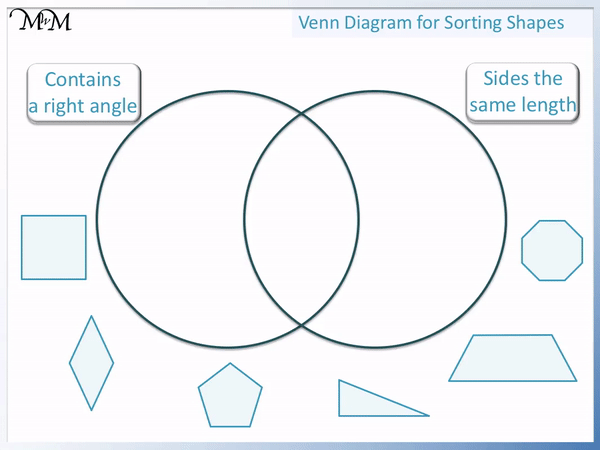• The rhombus (diamond) does not contain a right angle. All of its sides are the same length. It belongs in the right circle only.
• The pentagon does not contain a right angle. Its sides are all the same length. It too belongs in the right circle only.
• The triangle contains a right angle. Its sides are not all the same length. It belongs in the left circle only.
• The trapezium does not contain a right angle and all of its sides are not the same length. It belongs outside both circles.
• The octagon does not contain a right angle but its sides are all the same length. It belongs in the right circle only.

Here is another example of sorting shapes on a Venn diagram.

This time the left circle is for shapes with more than 3 sides and the right circle is for shapes that contain a parallel set of sides.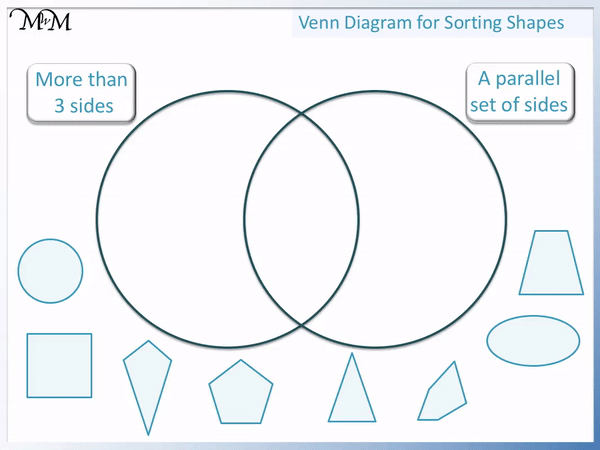• The circle does not have more than 3 sides and it does not have parallel sides. It belongs outside of both circles.
• The square has more than 3 sides and it has parallel sides. It belongs in the overlapping area of both circles.
• The kite has more than 3 sides but it does not have parallel sides. It belongs in the left circle only.
• The pentagon has more than 3 sides but it does not have parallel sides. It belongs in the left circle only.
• The triangle has 3 sides. This is not more than 3 sides. It does not have parallel sides. It belongs outside of both circes.
• The irregular pentagon has more than 3 sides and it does not have parallel sides. It belongs in the left circle only.
• The ellipse (oval) does not have more than 3 sides and it does not have parallel sides. It belongs outside of both circles on the Venn diagram.
• The trapezium has more than 3 sides and it has a set of parallel sides. It belongs in the overlap of both circles.Now try our lesson on Names of 3D Shapes where we learn the names of various common 3D shapes.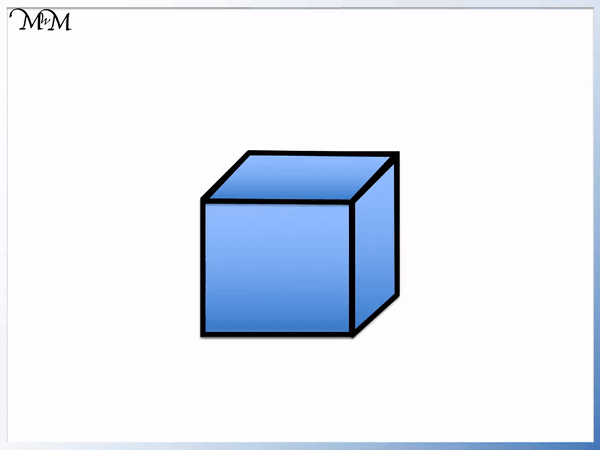error: Content is protected !!